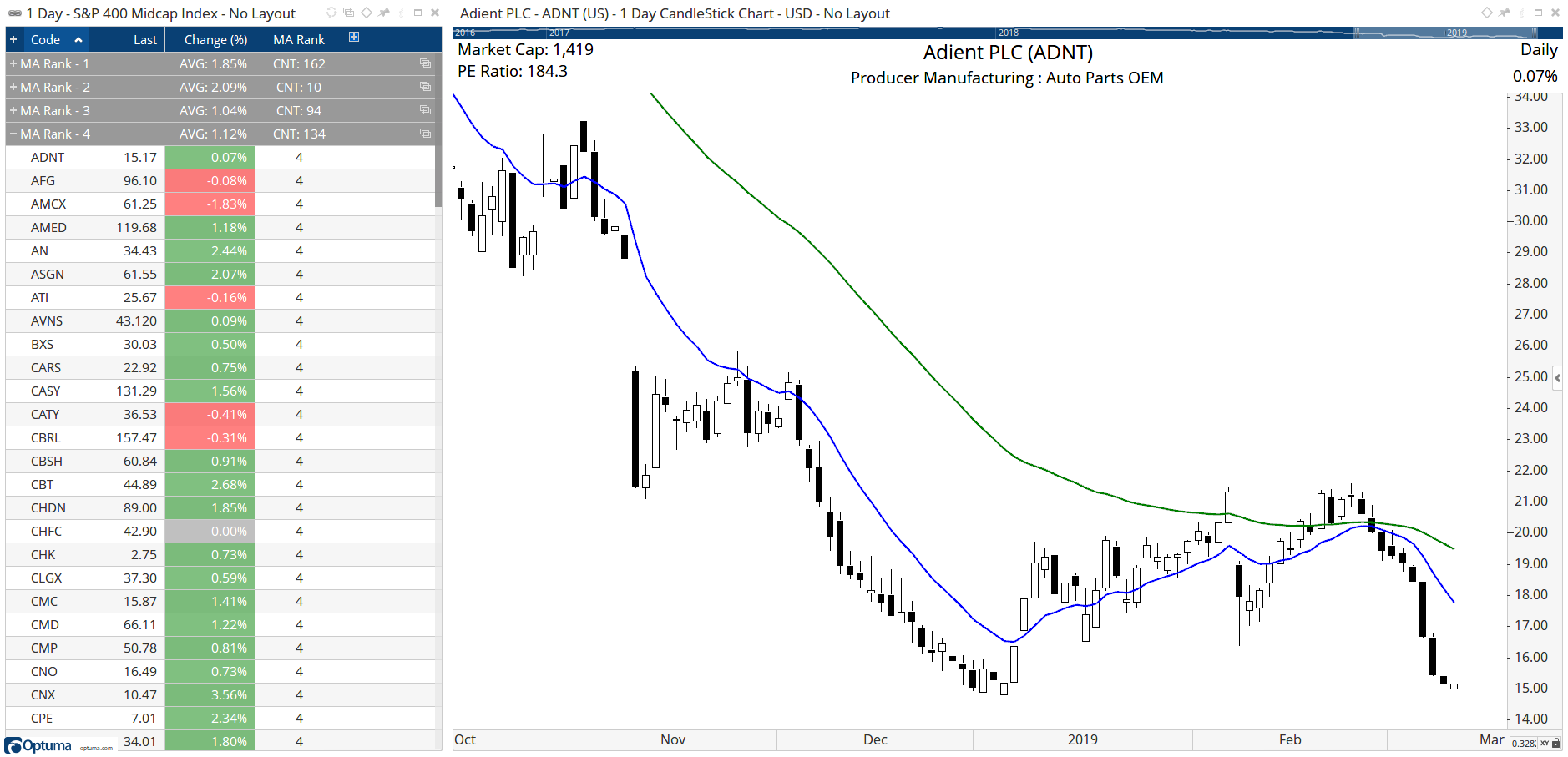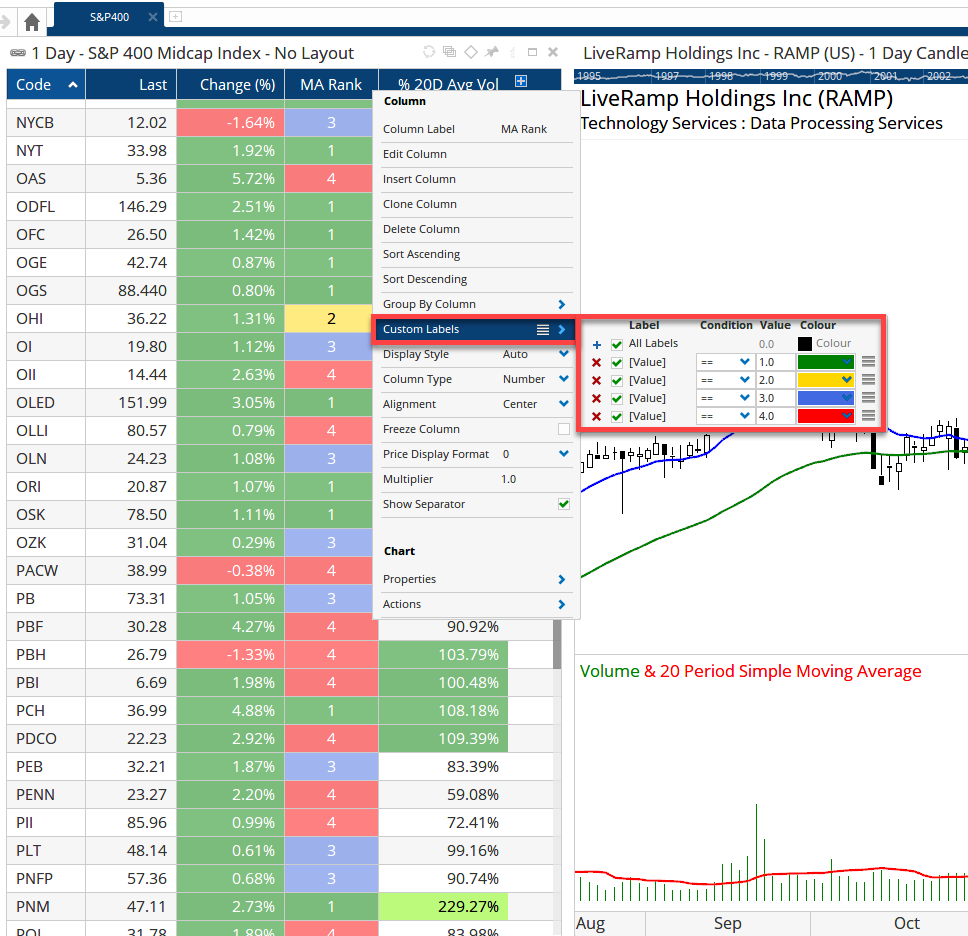Select Page

# Using the IF function to create a ranking value

Optuma Forums Optuma Scripting Using the IF function to create a ranking value

Viewing 5 posts - 1 through 5 (of 5 total)
• Author
Posts
• #28711

Here’s an example of using the IF() function to rank a stock according to a technical indicator value. For those of you familiar with Excel, the IF statement is based on the same principle as it introduces decision-making in to the formula. If the condition is true then one value is given, or if it is false then another is given. The syntax used is IF(formula, true value, false value).

For example, IF (CLOSE()> MA(BARS=50),CLOSE(), MA(BARS=50)) will return the closing price if it is higher than the 50 day moving average (i.e. the true condition), or the moving average if it is lower (i.e. the condition is false).

Note that if the true and false values are not specified (i.e just the IF(CLOSE() > MA(BARS=50)) then it turns in to a true/false Boolean condition, with a value of 1 given to a true result, and zero for false. To have a green True or red False result in a column rather than a value simply use CLOSE() > MA(BARS=50) without using the IF function.

In this example the following values will be assigned to each symbol based on whether two moving averages are sloping up or not:

1 = 13 Exponential MA and 50EMA both sloping up (condition 1 is true, and condition 2 is true)
2 = 13EMA sloping up and 50EMA sloping down (true, false)
3 = 13EMA sloping down and 50EMA sloping up (false, true)
4 = 13EMA and 50EMA both sloping down (false, false)

This can be expressed in the Optuma Scripting Language as follows:

This states that if the variable MA1 (the 13EMA) is sloping up (i.e. true) it will then look at variable MA2 (50EMA) and if that is also true a value of 1 will be assigned, but if MA2 is down then it will be assigned a 2 (false).

If the initial MA1 IsUp condition is false (ie it’s sloping down) then it will look to the second nested IF statement to determine whether MA2 IsUp is true (3) or false (4).

When added to a custom column in a Watchlist the values will be calculated for each symbol – as verified by the 13EMA Up? and 50EMA Up? columns in the attached image.

For clients with a Professional or Enterprise Services subscription these values can then be grouped, and summary counts / averages applied to them. In this example of the S&P400 Midcap Index 162 members have a MA Rank value of 1, 10 with a 2, 94 with a 3, and 134 where both EMAs are sloping down:#51587

Using the IF statement above to calculated a ranking value of the EMA slopes in a watchlist, it’s possible to colour the watchlist label based on the value colour the watchlist label based on the value:#67213

Hi. I’d like to build on the idea of ranking using IF statements in a watchlist, if possible.

I have 2 IF statements. They both work. Each IF statement assigns a number if true and a different number if false. I want to use them together to save space on my watchlist. Below I am pasting 2 scripts, but in reality the IF statements are in different scripts/columns:

The idea is to have 1 column in the watchlist instead of 2 columns. I want to see 4 if it’s an all time closing high, 3 if its a 252 bar closing high, 2 if it’s a 63 bar closing high, and 1 if it’s a 21 bar closing high. I have tried many, may things and cannot seem to crack the code. Please let me know if I can accomplish this.

thank you,
Louis

#67241

Hi Louis you need to nest all the IF statements together, as in my example in the first post.

However, because your ranking system isn’t mutually exclusive (i.e. if v4 is true then all the others must be true as well) then you have to start with V4 first and work backwards.

#67249

Thank you, Darren. This worked perfectly!

Viewing 5 posts - 1 through 5 (of 5 total)
• You must be logged in to reply to this topic.